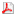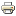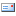### Product Manuals

 Math Parser FunctionsWritten by Phil Tobin
Saturday, 24 August 2013 10:03

The following functions are available for use in TunerStudio and MegaLog Viewer:

 Function Definition Usage Smoothing* Smooths a field by averaging over the smoothingFactor number of records. smoothBasic( field, smoothingFactor) sine Standard Sine of a value. sin(val) cosine Standard Cosine of a value. cos(val) arcsine Standard arcsine of a value. asin(val) arccosine Standard arccosine of a value. acos(val) tangent Standard Tangent of a value. tan(val) arc tangent Standard Arc Tangent of a value. atan(val) square root Standard Square Root, same as pow(val, 0.5) of a value. sqrt(val) absolute Changes any negative values to the same magnitude in the positive direction. abs(val) log Natural log of a value. log(val) log base 10 Base 10 log of a value of a value. log10(val) recipricol The recipricol of a value, or 1/val recip(val) exponent Exponent of a value. pow(val, exponent) round Rounds the value of a value to the nearest integer value. round(val) floor Returns the largest (closest to positive infinity) integer value that is greater than or equal to the argument. floor(val) ceiling Returns the smallest (closest to negative infinity) integer value that is greater than or equal to the argument. ceil(val) exponent Returns Euler's number e raised to the power of a double value. exp(val) isNaN Checks the output of an expression to result in a invalid number isNaN(val) smoothFiltered Smooth a field using a matrix filter for less lag smoothFiltered(val) accelHp Calculates HP based on Acceleration rate. accelHp( velocity (MPH), deltaVelocity(MPH), deltaTime(s), weight(lb) ) Aero Drag Calculates the Aerodynamic Drag aerodynamicDragHp( velocity (m/s), airDensity (kg/m^-3), dragCoefficent, frontalArea (m^2) ) Rolling Drag Calculates estimated rolling resistence rollingDragHp( speed (MPH), tirePressure (psi), weight (lbs) ) Last Value Returns the last record value for the specified field or expression lastValue(anyField) Historical Value Returns the value for the specified field or expression from n records back historicalValue(anyField, n) Min Returns the minimum value resulting from 1-n expressions or variables min(exp, exp, ...) Max Returns the maximum value resulting from 1-n expressions or variables max(exp, exp, ...) Max Value Returns the maximum historical value for the specified field or expression maxValue(anyField) Min Value Returns the minimum historical value for the specified field or expression minValue(anyField) table Perform a table lookup using an inc file table(expression, 'fileName.inc') tableLookup Perform a table lookup on 1D or 2D arrays based on specified OutputChannel(s) and 1D array lookup references. It will provide the interpolated value for the relative position of the lookup. tableLookup([array.valueArrayName], [array.lookupArrayName], [lookupChannelName])   tableLookup([array.zParamName], [array.xParamName], [array.yParamName], [xChannelName], [yChannelName]) Accumulate Accumulate and sum the expressionThis allows totalling distance, milage, fuel consumption, etc. accumulate(expression) Persistent Accumulate Same as accumulate, except the accumulated value is persisted to the next  session. Allows for an Odometer. persistentAccumulate(expression) arrayValue* Gets the interpolated value from a Constant array. The right of the decimal for index expression will be used for interpolation arrayValue(Constant, indexExpresssion) if* Excel style if function. if(condition, then, else) sum* Excel style sum function. sum(exp0, exp1, expN...) timeToExceed* Resets timer when expression is <= 0Once expression resolves to > 0, the timer begins and will count time in seconds until expression >= targetVal expression.Time expression is optional, if not provided the local clock is used. Thus in MLV it is required if you wish to use the Log time. timeToExceed(targetVal, expression, TimeExpression[optional])   Example for 0-60 in MLV:timeToExceed(60, [VSS1], [Time]) timeTrue* Evaluates the TrueFalseExpression and begins tracking the time it has been true (non-zero) in seconds. Once it is false, the time resets. timeTrue([TrueFalseExpression])   Example for 3 seconds after RPM > 600timeTrue( rpm > 600) timeTrueFor* Evaluates the TrueFalseExpression, will return false (0) until this expression evaluates as non-zero consistently for the period provided in seconds. isTrueFor([TrueFalseExpression], [SecondsTrueExpression]) Example: Will return 0 until rpm over 600 for 5 secondsisTrueFor( rpm > 600, 5)

*TunerStudio 3.1.03+ required.

Last Updated on Thursday, 02 April 2020 12:35

### Polls

What would you like to see in TunerStudio 3.0
 Integrated firmware loader Review Mode GPS Mapping with scatter plot overlay Integrated Log Viewer Remote tuning & logging More Dashboard enhancements Additional Navigation menu options, i.e. tree view Dyno / Tuning Views Trim Table Auto tune (1WB/cyl required) Paging difference reports (currently only shows 12 dialogs) MAF Calibration Analyzer Data log Sound and video in parallel to MS data logs.